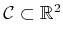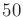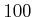## 8.2.3 Grid-Based Navigation Functions for Motion Planning

To consider feedback plans for continuous spaces, vector fields and other basic definitions from differential geometry will be needed. These will be covered in Section 8.3; however, before handling such complications, we first will describe how to use the ideas presented so far in Section 8.2 as a discrete approximation to feedback motion planning.

Examples 8.1 and 8.2 have already defined feedback plans and navigation functions for 2D grids that contain obstacles. Imagine that this model is used to approximate a motion planning problem for which. Section 5.4.2 showed how to make a topological graph that approximates the motion planning problem with a grid of samples. The motions used in Example 8.1 correspond to the 1-neighborhood definition, (5.37). This idea was further refined in Section 7.7.1 to model approximate optimal motion planning by moving on a grid; see Formulation 7.4. By choosing the Manhattan motion model, as defined in Example 7.4, a grid with the same motions considered in Example 8.1 is produced.

To construct a navigation function that may be useful in mobile robotics, a high-resolution (e.g.,topoints per axis) grid is usually required. In Section 5.4.2, only a few points per axis were needed because feedback was not assumed. It was possible in some instances to find a collision-free path by investigating only a few points per axis. During the execution of a feedback plan, it is assumed that the future states of the robot are not necessarily predictable. Wherever the robot may end up, the navigation function in combination with the local operator must produce the appropriate action. If the current state (or configuration) is approximated by a grid, then it is important to reduce the approximation error as much as possible. This is accomplished by setting the grid resolution high. In the feedback case, the grid can be viewed as covering'' the whole configuration space, whereas in Section 5.4.2 the grid only represented a topological graph of paths that cut across the space.8.3

Subsections
Steven M LaValle 2020-08-14